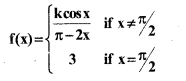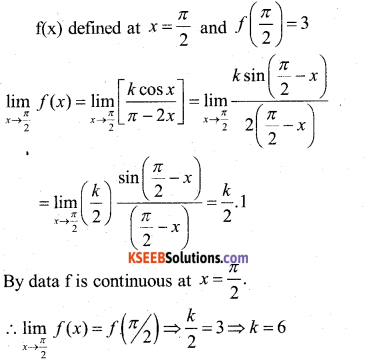# 2nd PUC Maths Model Question Paper 2 with Answers

Students can Download 2nd PUC Maths Model Question Paper 2 with Answers, Karnataka 2nd PUC Maths Model Question Papers with Answers helps you to revise the complete Karnataka State Board Syllabus and score more marks in your examinations.

## Karnataka 2nd PUC Maths Model Question Paper 2 with Answers

Time: 3 Hrs 15 Min
Max. Marks: 100

Instructions:

1. The question paper has five parts namely A, B, C, D and E. Answer all the parts.
2. Use the graph sheet for the question on Linear programming in PART E.

Part – A

I. Answer all the questions ( 10 × 1 =10 )

Question 1.
A Relation R on A = {1,2,3} defined by R = {(1,1) (1,2) (3,3)} is not symmetric, Why?
The Relation R is defined by R = {.(1,1) (1,2) (3,3)} for Symmetric Relation if (1,2)∈R then (2,1) ∈R but (2,1) ∉ R?
∴ R is not Symmetric Relation.

Question 2.
Write the domain of f(x) = cos-1 x.
The domain of cos-1x is [-1,1]

Question 3.
Define a scalar matrix.
A diagonal matrix in which all the Principle diagonal Elements are equal is called scalar matrix.Question 4.
If A = $$\left[ \begin{matrix} 1 & 2 \\ 3 & 4 \end{matrix} \right]$$ find |2A|.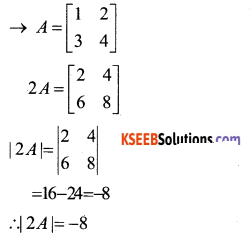Question 5.
If y = log (sin x) find $$\frac{\mathrm{dy}}{\mathrm{d} \mathrm{x}}$$
y = log(sin x)
Differentiae w.r.t.x, we get
$$\frac{\mathrm{dy}}{\mathrm{d} \mathrm{x}}$$ = $$\frac{1}{\sin x}$$ . cosx = cotx
$$\frac{\mathrm{dy}}{\mathrm{d} \mathrm{x}}$$ = cot x

Question 6.
Evaluate ∫( sin x + cos x) .dx.
∫(sinx + cosx).dx
= ∫ sin x.dx + ∫ cos x.dx.
= -cosx + sin x + c
= sinx – cosx + cQuestion 7.
Find the direction cosines of the vector $$\hat{\mathbf{i}}+2 \hat{\mathbf{j}}+3 \hat{\mathbf{k}}$$
$$\vec{a}=\hat{i}+2 \hat{j}+3 \hat{k}$$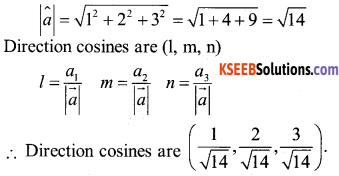Question 8.
Find the equation of the plane with intercept 4 on z-axis and parallel to xoy plane.
∴ The Required Equation of the plane is z =4.

Question9.
Define feasible region.
The Common Region determined by all the cons traints including the noh-negative constraints (x ≥ 0, y ≥ 0) of a linear programming problem is called the feasible region.

Question 10.
If P(A) = $$\frac{4}{5}$$ and P(B/A) = $$\frac{2}{5}$$ find
P(A∩B)
P(A /B) = $$\frac{P(A \cap B)}{P(A)}$$
∴ P(A∩B)  = P(B/A).P(A)
= $$\frac{2}{5} \cdot \frac{4}{5}=\frac{8}{25}$$
∴ P(A∩B) = $$\frac{8}{25}$$Part – B

II. Answer any TEN questions : ( 10 × 2 = 20 )

Question 11.
Verify whether the operation * defined on Q by a*b = $$\frac{\mathrm{ab}}{2}$$ is associative or not.
* is defined on Q by a*b = $$\frac{\mathrm{ab}}{2}$$ for associative we have to prove that
a* (b* c) = (a* b)* c
∴ a*(b*c) = a * $$\frac{\mathrm{ab}}{2}$$ b*c = $$\frac{\mathrm{bc}}{2}$$
= $$\frac{\mathrm{abc}}{4}$$ ……………….. (1)
(a*b)*c = $$\frac{\mathrm{ab}}{2}$$ *c
= $$\frac{\mathrm{abc}}{4}$$ ……………….. (2)
∴ from (1) and (2)
∴ * is Associative
∴ * Satisfies the associative property.

Question 12.
Write the simplest form of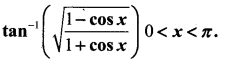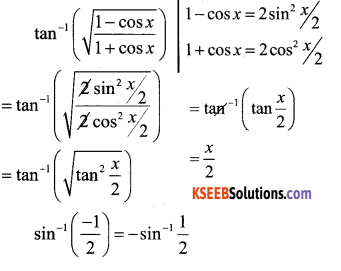Question 13.
Evaluate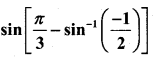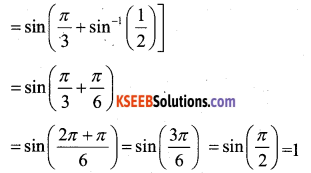Question 14.
Find the equation of line joining (1,2) and (3,6) using determinants.
Let P (x,y) be any point on AB. Then Area of triangle ABP is is “O”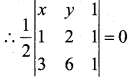x(2 – 6) – y(1 – 3) + 1(6 – 6) = 0
-4x + 2y = 0
2x – y = 0Question 15.
If y + siny = cosx find $$\frac{d y}{d x}$$
y + siny = cos x
Differentiate w.r.t. x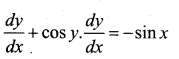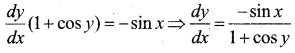Question 16.
If y = xx find $$\frac{d y}{d x}$$.
y = xx
Take logarithm on Both side, we get
logy = logxx ⇒ logy=xlogx
Differentiate wr.t. x
$$\frac{1}{y} \cdot \frac{d y}{d x}$$ = x x $$\frac{1}{x}$$ + log x
$$\frac{d y}{d x}$$ = y[1 + logx]
$$\frac{d y}{d x}$$ = xx[1 + logx]

Question 17.
Find the approximate charge in the volume v of a cube of side x metres caused by increasing the sind by 2%.
w.r.t volume of a cube = v = x3.
⇒ $$\frac{d v}{d x}$$ = 3x2 dx
Δx = 0.02 x
∴ dv = ( $$\frac{d v}{d x}$$ ) Δx = (3x2) Δx = 3x2 x 0.02x
= 0.06 x3m3Question 18.
Evaluate $$\int \frac{\sin ^{2} x}{1+\cos x} d x$$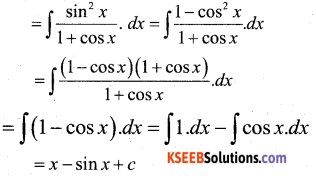Question 19.
Evaluate $$\int_{1}^{e} \frac{1}{x} \cdot d x$$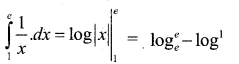Question 20.
Find the order and degree of the differential equation.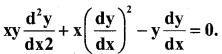Order -2, Degree – 1

Question 21.
If $$\overrightarrow{\mathrm{a}}$$ is a Unit Vector such that
( $$\vec{x}-\vec{a})(\vec{x}+\vec{a}$$) = 8 find  | $$\overrightarrow{\mathbf{x}}$$ )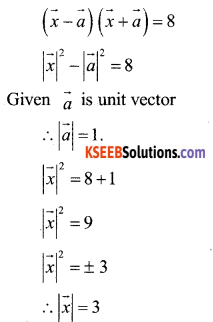Question 22.
Find the area of a parallelogram whose adjacent sides are given by the vectors
$$\overrightarrow{\mathrm{a}}=3 \hat{\mathrm{i}}+\hat{\mathrm{j}}+4 \hat{\mathrm{k}} \cdot \overrightarrow{\mathrm{b}}=\hat{\mathrm{i}}-\hat{\mathrm{j}}+\hat{\mathrm{k}}$$
The area of a parallelogram with $$\vec{a}$$ and
$$\vec{b}$$ as its adjacent sides is given by | $$\vec{a} \times \vec{b}$$ |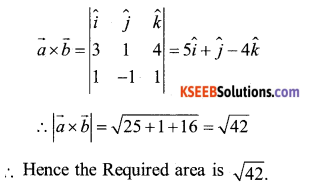Question 23.
Find the angle between the pair of lines.
$$\vec{r}$$ = 3î + 2 ĵ – 4k̂ + λ (î + 2ĵ + 2k̂) and
$$\vec{r}$$ = 5î + 2 ĵ + λ (3î + 2 ĵ + 6k̂)
Here
$$\vec{b}_{1}$$ = î + 2 ĵ + 2k̂ and  $$\vec{b}_{1}$$ = 3î + 2 ĵ + 6k̂
The angle θ between the two lines is given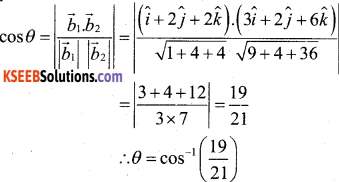Question 24.
Find the probability ditribution of number of heads in two tosses of a coin.
When a coin is tossed twice the sample space is S = {HH,HT, TH,TT}
Let x denote the number of heads in any out come in s.
∴x(HH) = 2
x(HT) = 1
x(TH) = 1
x(TT) = 0
P(x = 0) = P(TT) = $$\frac{1}{4}$$
P(x = 1) P(HT,TH) = $$\frac{2}{4}$$ = $$\frac{1}{2}$$
P(x = 2) P(HT,TH) = $$\frac{1}{4}$$
Probability distribution of x is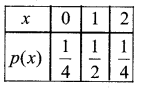Part – C

III. Answer any TEN questions : ( 10 × 3 = 30 )

Question 25.
Find gof and fog if 1:R → R and g: R → R are given by f(x) = cosx and g(x) = 3x2 show that gof ≠ fog.
f(x) = cosx
g(x)=3x2
gof(x) = g[f(x)]
= g[cosx]
= 3cos2x
fog(x) = f[g(x)]
= f[3x2]
= cos3x2
fog ≠ gof

Question 26.
P.T. 3cos-1x=cos-1(4x3 – 3x) x ∈ $$\frac{1}{2}$$ , 1
cos-1 x = A Now $$\frac{1}{2}$$ ≤ x ≤ 1
cosA = x
cos3A = 4cos3 A – 3cosA ⇒$$\frac{1}{2}$$ ≤ A < 1
cos3A=4x3 – 3x
3A = cos -1(4x3 – 3x) ⇒ 0 ≤ A ≤ $$\frac{\pi}{3}$$
3cos-1x = cos-1 [4x3 – 3x] ⇒ 0 ≤ 3A ≤ π

Question 27.
By using Elementary transformations
find the iii,e of the matrix
A = missing
In order to use elementary row operation
A = IA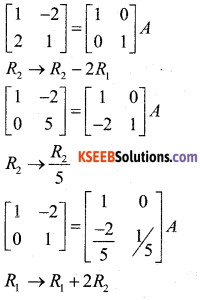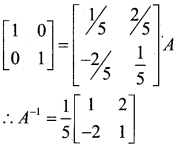Question 28.
Find $$\frac{d y}{d x}$$ if x = a(cosθ + θsinθ) and dx
y = a(sinθ – θcosθ)
x = a(cosθ + θsinθ)
Diff w.r.t θ we have
$$\frac{d x}{d \theta}$$ = a(-sinθ + θcosθ + sinθ )
$$\frac{d x}{d \theta}$$ = a[θcosθ]
y = a(sinθ – θcosθ)
w.r.t θ
$$\frac{d y}{d \theta}$$
= a[cosθ – θ x sin θ – cosθ]
$$\frac{d y}{d \theta}$$ = a[sinθ]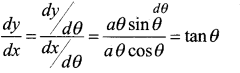∴ $$\frac{d y}{d x}$$ = tan θQuestion 29.
Verify Rolle’s theorem for the function y = x2 + 2, x ∈[-2,2]
The function y = x2 +2 is continuous in [-2,2], and differentiable in (-2,2)
Also f (-2) ⇒ f (x) = x2 + 2
f(- 2) = (-2)2 + 2
= 4 + 2 = 6
f(2) = 22 + 2 = 6
∴ f(-2) = f(2) = 6
∴ The value of f(x) at -2 and 2 coincide.
∴ Rolle’s Theorem states that there is a point
c ∈ (-2,2) where f'(c) = 0
Since f'(x) = 2x, we have
f’ (c) = 2c
2c = 0
c = 0
∴ we have c = 0 ∈ (-2,-2)

Question 30.
Find the interval in which the function f given by f (x) = x2 – 4x + 6 is
(i) Strictly increasing.
(ii) Strictly decreasing.
f(x) = x2 – 4x + 6
Differentiate w.r.t x
f'(x) = 2x – 4
∴ f'(x) = 0 gives
0 = 2x – 4
2x = 4
x = 2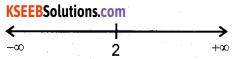∴ The point x = 2 divides the real line into two disjoint intervas namely, (-∞,2) and (2,∞).
The interval (-∞, 2) f'(x) = 2x – 4 < 0.
∴ f is strictly decreasing in (-∞, 2). Also in the interval (2,∞) f'(x)>0 and so the function f is strictly increasing in (2,∞).

Question 31.
Find $$\int \frac{\left(x^{2}+1\right) \cdot e^{x}}{(x+1)^{2}} \cdot d x$$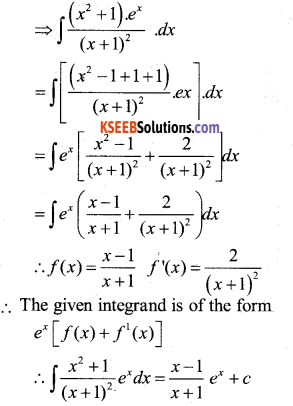Question 32.
Evaluate ∫tan -1x.dx.
∫tan -1x.dx.
= ∫xtan -1x. ∫tan -1dx + c
Apply Rule of integration by parts by taking tan-1 x as the first function.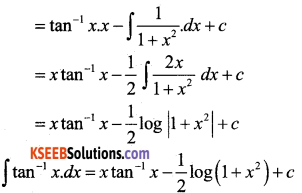Question 33.
Find the area of the region bounded by the curve y = x2 and the line y = 4.
y = x2 represents the parabola symmetrical about y – axis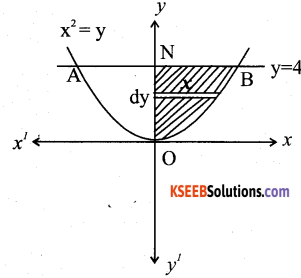∴ The Area of the region AOBA is given by 2 $$\int_{0}^{4} x \cdot d y=2$$ [Area of the region BONB bounded by curve, y-axis and the lines y=0 and y=4]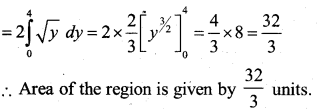Question 34.
Form the differential equation representing the family of curves y=mx where m is arbitrary constant.
The given family of curves represents the equation of straight lines.
∴ y = mx ……………………. (1)
Differentiate w.r.t x, we get dy
$$\frac{d y}{d x}$$ = m
∴ substitute m = $$\frac{d y}{d x}$$, we have
y = mx
⇒ y = $$\frac{d y}{d x}$$ x
$$\frac{d y}{d x}$$ = $$\frac{y}{x}$$
$$\frac{d y}{d x} – \frac{y}{x}$$ = 0
which is the required differential equation.

Question 35.
Prove that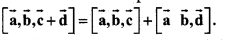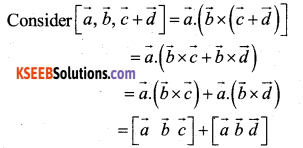Question 36.
If $$\vec{a}=2 \vec{i}+2 \hat{j}+3 \hat{k} \overrightarrow{\mathbf{b}}=-\hat{\mathbf{i}}+2 \hat{\mathbf{j}}+\hat{\mathbf{k}} and [latex]\overrightarrow{\mathbf{c}}=3 \hat{\mathbf{i}}+\hat{\mathbf{j}}$$  are such that $$\overrightarrow{\mathbf{a}}+\lambda \overrightarrow{\mathbf{b}}$$ is perpendicular to $$\overrightarrow{\mathrm{c}}$$. Then find the value of λ.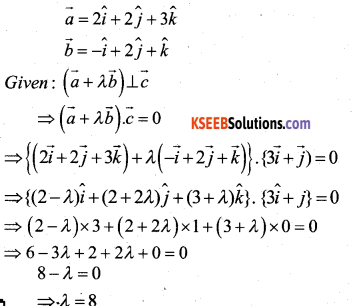Question 37.
Find the distance of a point (2, 5, -3) from the plane $$\vec{r} \cdot(6 \hat{i}-3 \hat{j}+2 \hat{k})=4$$
$$\vec{a}=2 \hat{i}+5 \hat{j}-3 \hat{k}$$
$$\vec{N}=6 \hat{i}-3 \hat{j}+2 \hat{k}$$
d = 4
distance of a point from a plane is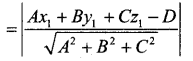∴ The distance of the point (2, 5 – 3) from the
given plane is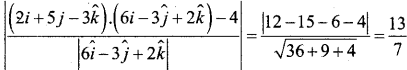Question 38.
A die is tossed thrice. Find the probability of getting an odd number at least once.
When a dice is tossed thrice, the sample space, is S = {(x,y,z):x,y z e (1,2,3,4,5,6}}
which contains 6 x 6 x 6 = 216 Equally likely sample points.
Let E : “an odd number at least once”
E1 : not an odd number any time
E1 : “An even number on all throws”
E1 = {(x,y,z):x,y,ze{2,4,6}j
e1 contains 3x3x3=27 equally likely simple events.
Required probability = P(E) = 1 – P(E).
= $$1-\frac{27}{216}=1-\frac{1}{8}=\frac{7}{8}$$Part – D

IV. Answer any SIX questions : ( 6 × 5 =30 )

Question 39.
Prove the function f : N → Y defined by f(x) = 4x + 3, where Y = {y : Y = 4x + 3, x∈N} is invertible. Also write the inverse of f(x).
f:N→y defined by
f(x) = 4x + 3
and Assume a an inverse f-1:y → N. is defined by f-12y) = x.
∴ wk.t
f(x) = 4x + 3
y = 4x + 3
y – 3 = 4x
x = $$\frac{y-3}{4}$$
and wk 7
f-1(y) = x
f-1(y) = $$\frac{y-3}{4}$$ f-1 = g
or
g(y) = $$\frac{y-3}{4}$$
∴ To S.T. it exist inverse.
To prove it is inversible.
We have to prove that
fog(x) = x
gof(y) = y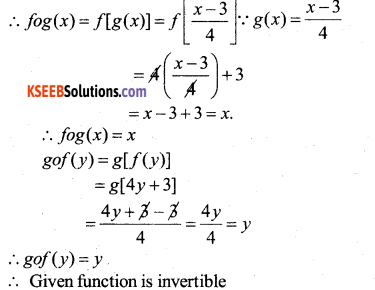To find the inverse of a function :
f-1:y → N. by f-1(y) = x
f-1(y) = $$\frac{y-3}{4}$$

Question 40.
If A = $$\left[ \begin{matrix} 1 & 2 \\ 2 & 1 \end{matrix} \right]$$ B = $$\left[ \begin{matrix} 2 & 0 \\ 1 & 3 \end{matrix} \right]$$ and C = $$\left[ \begin{matrix} 1 & 1 \\ 2 & 3 \end{matrix} \right]$$ Calculate AC BC and (A+B).C. Also verify that (A+B). C = AC+BC.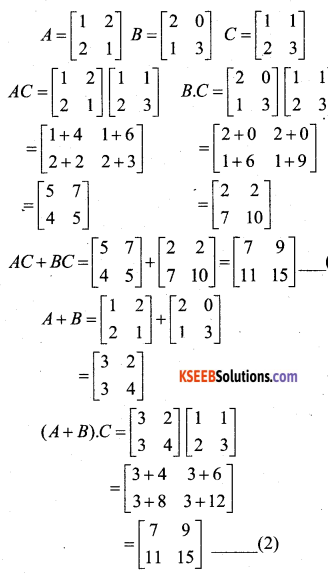From (1) and (2)
(A+B). C = AC+BC.

Question 41.
Solve the following system of equations by matrix method. x-y + z = 4, 2x + y – 3y = 0 andx + y + z =2.
The given system can be written as AX = B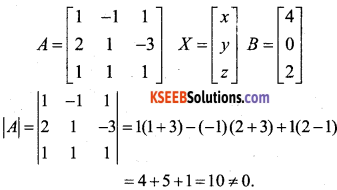The given system is consistent and has a unique Solution.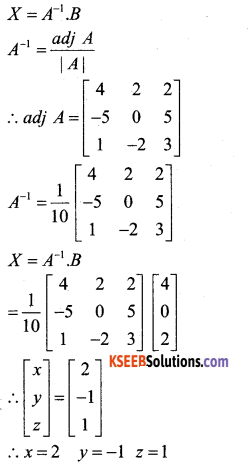Question 42.
If y = 3e2x+2e3x P.T. $$\frac{d^{2} y}{d x^{2}}$$ – $$\frac{d y}{d x}$$ + 6y = 0
y = 3e2x + 2e3x
Differentiate w.r.t x.
$$\frac{d y}{d x}$$ = 6e2x + 6e3x
Again Diff w.r.t x
$$\frac{d^{2} y}{d x^{2}}$$ = 12e2x + 18e3x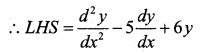= 12e2x +183x – 5 ((5e2x + 6e3x) + 6(3e2x + 2e3x)
= 12e2x + 183x – 30e2x – 30e3x +18e2x + 12e3x
= 30e2x + 30e3x – 30e2x – 30e3x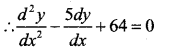Question 43.
A ladder 24 ft long leans against a vertical wall. The lower end is moving away at the rate of 3ft/sec. Find the rate at which the top of the ladder is moving down wars. If its foot is 8ft from the wall.
The foot of the ladder is at a distance x from the wall and the top is at a height y at instant of time t. then
(24)2 = x2 + y2
Diff w.r.t time, we get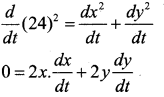given $$\frac{d x}{d t}$$ = 3ft / sec. dt
and x = 8 ft.
y2 = (24)2 – x2
= (24)2 – (8)2
= 576 – 64
y2 = 512
y = √512
0 = 2 × 8 × 3 + 2 × √5I2 $$\frac{d y}{d t}$$
= 48 + 2√512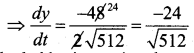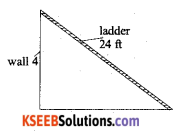∴ The ladder is moving downwards at rate of $$\frac{24}{\sqrt{512}}$$ ft/ sec. or 1.06100 ft / sec.

Question 44.
Find $$\int \frac{d x}{x^{2}-a^{2}}$$ and hence evaluate $$\int \frac{d x}{3 x^{2}+13 x-10}$$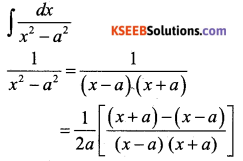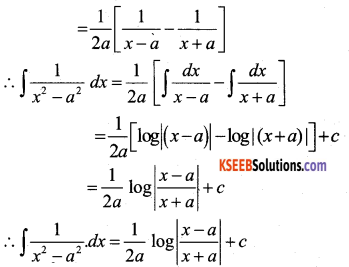Question 45.
Find the area of the region bounded by the two parabolas y = x2 and y2 = x.
The given two curves are parabola y = x2 and y2 = x
The point of intersection of these two parabolas are 0 (0,0) and A (1,1) as shown in the fig.
We can set
y2 =x or y = √x – f (x)
y – x2 = g(x) where
f(x) ≥ g(x) in [0,1]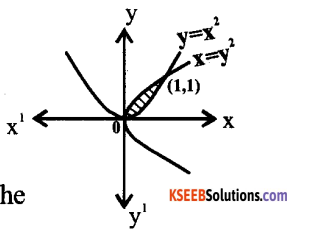∴ The required area of the Shaded Region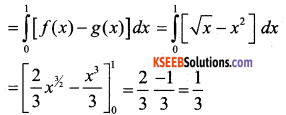∴The Required area of the Region is = $$\frac{1}{3}$$ units.Question 46.
Find the general solution of the differential equation. e/tany. dx + (1 -ex). sec2y.dy = 0
ex. tany dx + (1-ex). sec2 y dy = 0 (1)
Separating the variables and integrating, we get
This can be done by divide throughout (l-ex)t any Eqn. (1) becomes and integrate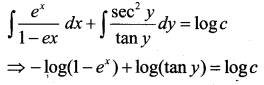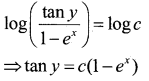Which is the required solution.

Question 47.
Derive the equation of the plane in normal form both in the cartesian and vector form.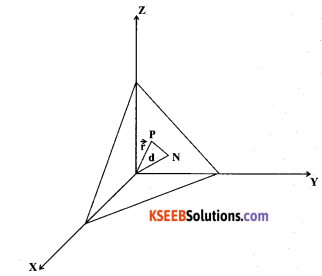Consider a plane whose perpendicular distance from the origin is d. where d ≠ 0.
If $$\overrightarrow{\mathrm{ON}}=d \hat{\mathrm{n}}$$
Let p be any point on the plane Therefore NP.OP=0(l)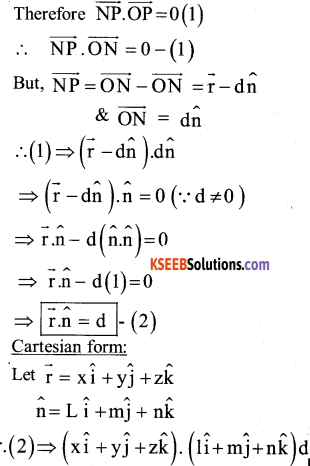lx + my + nz = d
This is the Required Equation of a plane in normal form.

To find P and Q :
x2 = 4y and x = 4y – 2
(4y – 2)2 = 4y
⇒ 16y2 + 4 – 16y = 4y
⇒ 16y2 – 20y + 4 =0
⇒ (y – 1) (16y – 4) =0
⇒  y =1 (or) y = 1/4
Ify=1; x = 2 ⇒ 2 (2,1)
and y = 1/4 x = -1 (-1, 1/4)
Thus , the points of Intersection are
(2,1) 1 (-1.,1/4)
∴ Area of the Region O B Q C = Area of BCQ+ Area of QCO.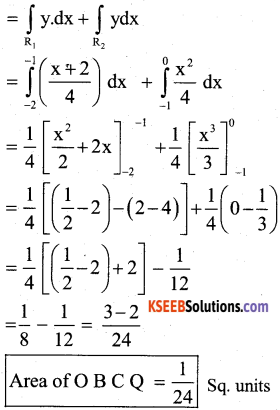Question 48.
A person buys a lottery ticket in 50 lotteries in each of which his chance of winning a prize is $$\frac{1}{100}$$ what is the probability that he will win a prize exactly once?
Given it is a lax of Bemoullian trials with n = 50 and P = $$\frac{1}{100}[latex] Hence q = 1 – P = 1 – [latex]\frac{1}{100}$$ = $$\frac{99}{100}$$
The Probability that he will win a prize exactly once is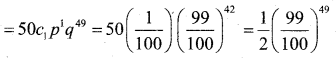Part – E

V. Answer any ONE questions : ( 1 × 10 = 10 )

Question 49.
a) Minimize and maximize Z = x + 2y subject to the constraints,
x + 2y ≥ 100
2x – y ≤ 0
2x + y ≤ 200
x, y ≥ 0 by graphical method.
Our problem is to minimize and maximize
Z = x + 2y …………..(1)
Subject to constraints are
x + 2y ≥ 100 …………….(2)
2 x – y ≤ 0 ……………..(3)
2x + y ≤ 200…………….(4)
x> 0, x>0…………….(5)
∴ x + 2y- 100 which passes through the points A( 100,0) and B(0,50)
2x – y = 0 which passes through the points (0,0) and (20,4 0)
2x + y = 200 which passes through the points A(100,0)&C (0,200)
∴ The comer points of feasible region are 5(0,50) P(20,40) 0(50,100) and C(0,200).
At B (0,50) ⇒ Z = 0 + 2 × 50 = 100
At P(20,40) ⇒ Z = 20 + 2 × 40 – 100
At Q(5O,1OO) ⇒ Z = 50 + 2 × 100 = 250
At C(0,200) Z = 0 + 2 × 200 = 400
Hence max Z = 400 at (0, 200) and
Min Z = 100 at B and also at P.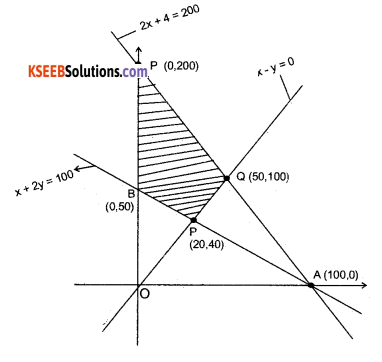(b)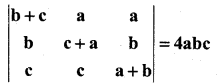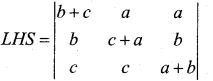R1 R1 – (R2 + R3)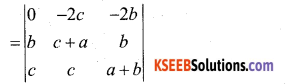= 0 [(c + a) (a + b) – bc) + 2c (b (a + b) – bc) – 2b (bc – (c + a)]
= 0 + 2c [ab + b2 -bc] – 2b [bc – c2 -ac]
= 2abc + 2cb2 – 2bc2 – 2b2c + 2bc2 + 2abc
= 4 abc = RHSQuestion 50.
a) Prove that $$\int_{0}^{a} f(x) \cdot d x=\int_{0}^{a} f(a-x) \cdot d x$$ and hence evaluate $$\int_{0}^{\pi / 4} \log (1+\tan x) \cdot d x$$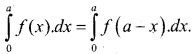put t = a- x
x = a – t
than dt = -dx
when x = 0 x = a
t = a t = 0
then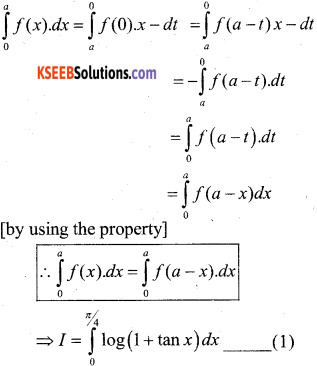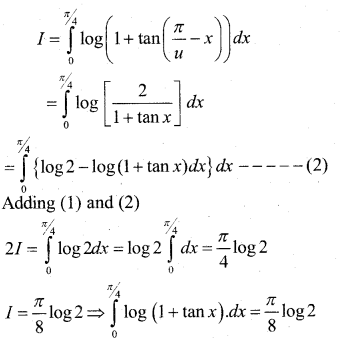(b) Find the value of k, if